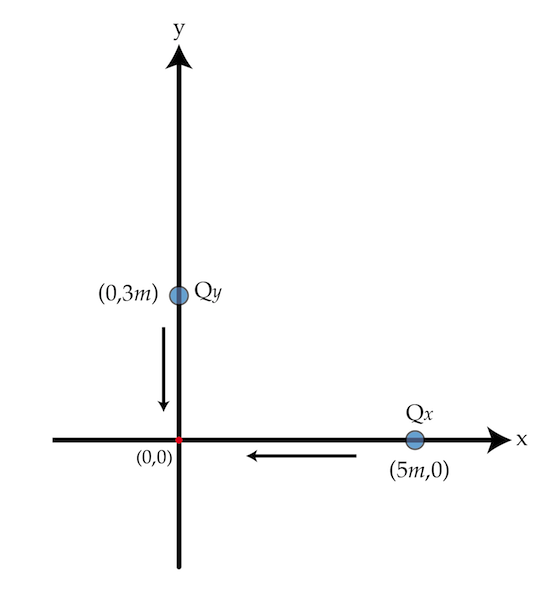Electricity and Magnetism

# E+M Warmups: Level 3 Challenges

Consider a small black ball of radius $R$ and density $\rho= 1~\frac{\mbox{g}}{\mbox{cm}^{3}}$ located at a certain distance above the surface of the Sun. For what radius $R$ in micrometers is the gravitational attraction of the Sun counterbalanced by the radiation force?

Assume that the black ball absorbs all the incident light and that the total power radiated by the Sun is $P=4\times 10^{26}~\mbox{W}$.

Details and assumptions

The mass of the Sun is $M_{s}=2\times 10^{30}~\mbox{kg}$. The universal constant of gravitation is $G=6.67\times 10^{-11}~\text{m}^{3}\text{kg}^{-1} \text{s}^{-2}$ and the speed of light $c=3\times 10^{8}~\mbox{m/s}$.

Two 1-g beads with charges $Q_{x}=2~\mu\mbox{C}$ and $Q_{y}=-3~\mu\mbox{C}$ slide on (without friction) two perpendicular wires ($x$ and $y$ axes). The charges are initially located at $(5~\mbox{m},0)$ and $(0,3~\mbox{m})$ and released from rest.What is the distance in meters between the charges when $Q_{y}$ crosses the origin $(0,0)$?

Details and assumptions

$k=\frac{1}{4\pi \epsilon_{0}}=9 \times 10^{9} \mbox{N m}^{2}/\mbox{C}^{2}$Three identical conducting spheres are located at the vertices of an equilateral triangle ABC. Initially the charge the charge of the sphere at point A is $q_{A}=0$ and the spheres at B and C carry the same charge $q_{B}=q_{C}=q.$ It is known that the sphere B exerts an electrostatic force on C which has a magnitude $F=4~\mbox{N}.$ Suppose an engineer connects a very thin conducting wire between spheres A and B. Then she removes the wire and connects it between spheres A and C. After these operations, what is the magnitude of the force of interaction in Newtons between B and C?

Christmas tree lights can be put together in either series or parallel. Consider 100 Christmas tree lights, each with a resistance of 1$\Omega$. Let circuit P be all the lights hooked up in parallel to an electrical outlet, and circuit S be all the lights hooked up in series. What is the ratio of the power used in P to the power used in S?

The Hyperloop is a hypothetical new fast transport system between cities, which works by launching pods that carry people through a very low air pressure tunnel. If we model the Hyperloop pod as a hollow metal cylinder with a radius of $1~\mbox{m}$ and a length of $10~\mbox{m}$, what is the maximum voltage difference between the sides of the pod in Volts?

Details and assumptions

• The magnetic field at the earth's surface is $0.5~\mbox{Gauss}$.
• The speed of the pod is $300~\mbox{m/s}$.
×

Problem Loading...

Note Loading...

Set Loading...